SSC BOARD PAPERS IMPORTANT TOPICS COVERED FOR BOARD EXAM 2024

### Practice Set 2.6 Quadratic Equations Class 10th Mathematics Part 1 MHB Solution

Practice Set 2.6

1. Product of Pragati’s age 2 years ago and 3 years hence is 84. Find her present age.…
2. The sum of squares of two consecutive natural numbers is 244; find the numbers.…
3. In the orange garden of Mr. Madhusudan there are 150 orange trees. The number of trees…
4. Vivek is older than Kishor by 5 years. The sum of the reciprocals of their ages is 1/6.…
5. Suyash scored 10 marks more in second test than that in the first. 5 times the score of…
6. Mr. Kasam runs a small business of making earthen pots. He makes certain number of pots…
7. Pratik takes 8 hours to travel 36 km downstream and return to the same spot. The speed…
8. Pintu takes 6 days more than those of Nishu to complete certain work. If they work…
9. If 460 is divided by a natural number, quotient is 6 more than five times the divisor…
10. In the adjoining fig. □ABCD is a trapezium AB||CD and its area is 33 cm^2 . From the…

###### Practice Set 2.6
Question 1.

Product of Pragati’s age 2 years ago and 3 years hence is 84. Find her present age.

Let her present age be x

According to question,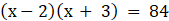⇒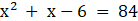⇒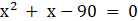⇒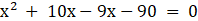⇒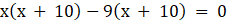⇒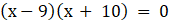⇒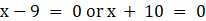⇒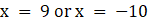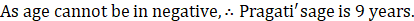Question 2.

The sum of squares of two consecutive natural numbers is 244; find the numbers.

Let the two consecutive natural numbers be x and x + 2. Then,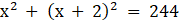⇒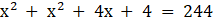⇒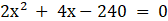⇒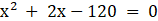⇒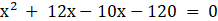⇒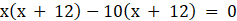⇒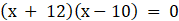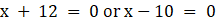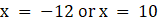No.s cannot be negative, ∴ numbers are 10 and 12

Question 3.

In the orange garden of Mr. Madhusudan there are 150 orange trees. The number of trees in each row is 5 more than that in each column. Find the number of trees in each row and each column with the help of following flow chart.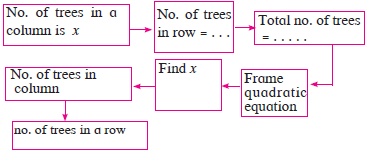Let the number of columns be x

∴ rows = x + 5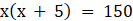⇒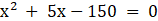⇒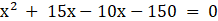⇒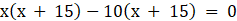⇒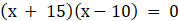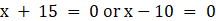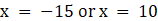Hence, columns cannot be negative. ∴ columns are 10

and rows are 15.

Question 4.

Vivek is older than Kishor by 5 years. The sum of the reciprocals of their ages is 1/6. Find their present ages.

Let Kishor’s present age be x. Then, vivek’s age = x + 5

∴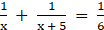⇒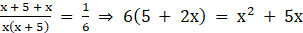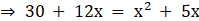⇒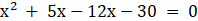⇒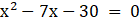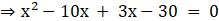⇒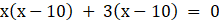⇒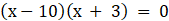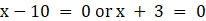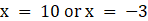Hence, age cannot be negative. ∴ age od Kishor is 10

and age of Vivek is 15.

Question 5.

Suyash scored 10 marks more in second test than that in the first. 5 times the score of the second test is the same as square of the score in the first test. Find his score in the first test.

Let the score of first test be x. Then, second test score = x + 10.

∴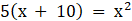⇒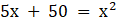⇒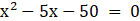⇒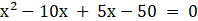⇒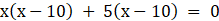⇒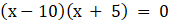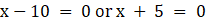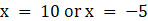Hence, score of first test is 10 as marks are not negative.

Question 6.

Mr. Kasam runs a small business of making earthen pots. He makes certain number of pots on daily basis. Production cost of each pot is ₹40 more than 10 times total number of pots, he makes in one day. If production cost of all pots per day is ` 600, find production cost of one pot and number of pots he makes per day.

Let the number of pots made by Mr. Kasam each day be x. Then, production cost of each pot =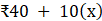∴ total cost =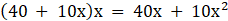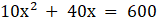⇒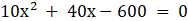⇒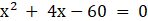⇒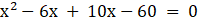⇒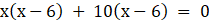⇒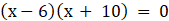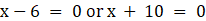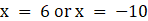Hence number of pots made cannot be negative. ∴ number of pots he made each day = 6

Cost of one pot =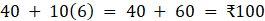Question 7.

Pratik takes 8 hours to travel 36 km downstream and return to the same spot. The speed of boat in still water is 12 km. per hour. Find the speed of water current.

Let the speed of water current be x.

∴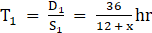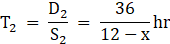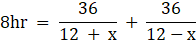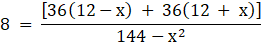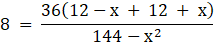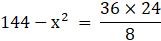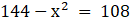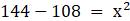⇒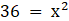⇒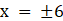Speed od water current is 6km/hr

Question 8.

Pintu takes 6 days more than those of Nishu to complete certain work. If they work together they finish it in 4 days. How many days would it take to complete the work if they work alone.

Suppose Nishu alone takes x days to finish work. Then , Pintu alone can finish in (x + 6)days.

⇒ Nishu’s one day work + Pintu’s one day work =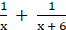(Nishu + Pintu)’s one day work =∴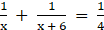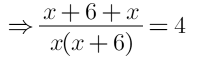⇒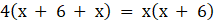⇒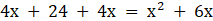⇒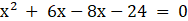⇒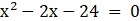⇒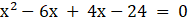⇒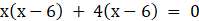⇒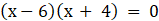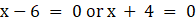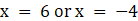x = -4 is not possible, as no of days can't be negative.

Nishu will take 6 days alone and Pintu takes 12 days alone.

Question 9.

If 460 is divided by a natural number, quotient is 6 more than five times the divisor and remainder is 1. Find quotient and diviser.

Let the divisor be x. Then, Quotient be 6 + 5x

Now according to question,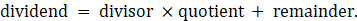⇒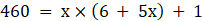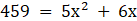⇒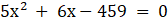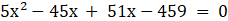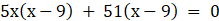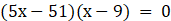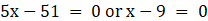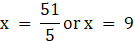∴ divisor = 9 and quotient =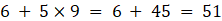∴Divisor = 9, quotient = 51

Question 10.

In the adjoining fig. □ABCD is a trapezium AB||CD and its area is 33 cm2. From the information given in the figure find the lengths of all sides of the □ABCD. Fill in the empty boxes to get the solution.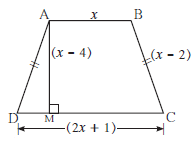□ ABCD is a trapezium.

AB||CD

A(□ABCD) =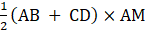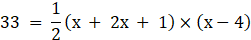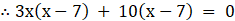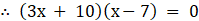∴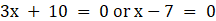∴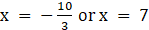But length is never negative.

∴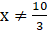∴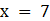AB = 7 cm, CD = 15 cm, AD = BC = 5 cm.